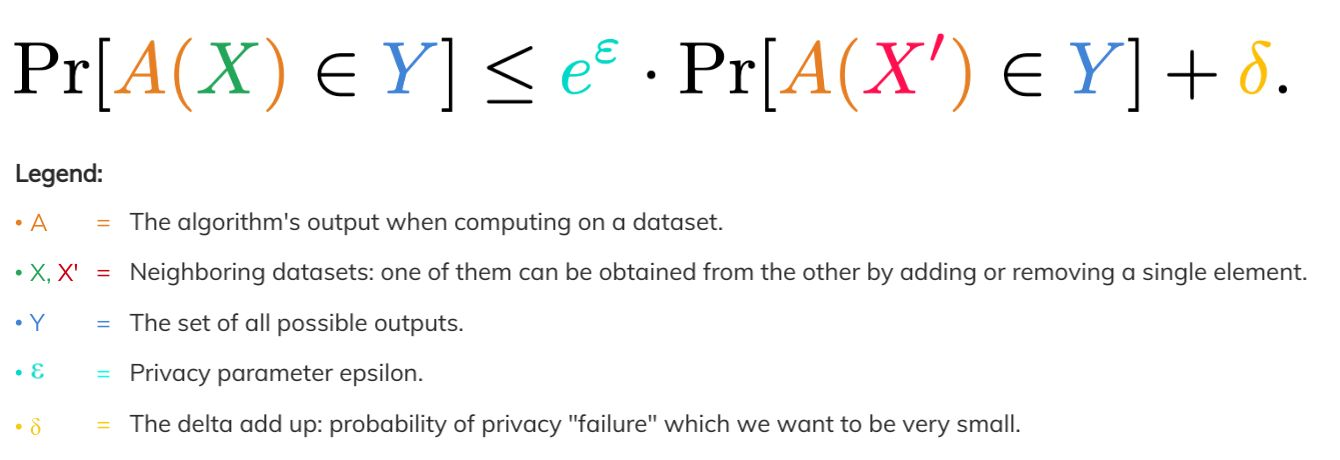### TL;DR?

Watch our short explainer video on Differential Privacy!

## Differential Privacy

Differential Privacy is not actually a technology, it can instead be viewed as a promise made by a data holder to a data subject and goes as follows:

“You will not be affected, adversely or otherwise, by allowing your data to be used in any study or analysis, no matter what other studies, data sets, or information sources, are available.”

(Algorithmic Foundations of Differential Privacy by Cynthia Dwork).

### Some Intuition

Another way of looking at Differential Privacy is as a contract made between an algorithm and an individual’s data record, guaranteeing that when applied on the dataset - the algorithm will produce an output with the same probability whether the given record was part of the computation or not. This means that from an individual’s perspective, the algorithm is obligated to not rely on her data in a way that will impact the outcome of the computation. Sounds too good to be true? In the world of big data this is actually quite trivial. Imagine an enterprise with millions of records in each dataset - why should a single record be that meaningful for any type of analytics? It wouldn’t. However, keep in mind that a regular algorithm, without a Differential Privacy “contract”, cannot guarantee privacy even if its output is an aggregated query result over all those millions of records. This is because the contribution of a specific person can be quantified from several such queries and allow an attacker to collapse to its target individual (read more about it on our blog post “Top 4 Data Privacy Misconceptions by Corporates”).

### Formal Definition

To uphold a Differential Privacy "contract", an algorithm must adhere to the following formula:Simply put, this means that the probability of algorithm A to produce an output from Y - whether applied on dataset X or its neighbor X’ - differs by just e^ε, plus a very small delta (for example, 2^(-n) where n is the size of the dataset). When δ=0, we say the algorithm satisfies pure Differential Privacy or: ε-differential privacy. Privacy parameter ε (or the privacy budget) of a Differential Privacy algorithm determines just how much noise needs to be introduced to the computation process in order to mask the contribution of every single individual, depending on the desired tradeoff between privacy and utility:

• When ε = 0, there is equal probability to get the output from both datasets - privacy is fully preserved with lower accuracy.
• When ε = ∞, privacy is fully compromised since a difference in a single record completely changes the output probability (we get higher accuracy at the expense of privacy).

### So, what exactly is this noise?

Let's look at an example: think of a dataset containing the ages of 100 participants in a medical experiment. Let’s assume the minimum possible age of a human is 0 years and the maximum possible age is 120 years. If the algorithm is supposed to compute the average age of a participant in the experiment, then regardless of any actual record in the dataset we can infer that a single record (removed or added) can, at most, influence the result by 120 divided by 100. This maximum possible distance in a query’s result when removing or adding an individual from the dataset is known as the sensitivity of the problem.Randomization, or noise, must be introduced into the algorithm's response in order to hide the participation of a single individual.

The sensitivity captures the magnitude by which a single individual’s data can change the output in the worst case, and therefore, intuitively, the randomization (or noise) that we must introduce into the response in order to hide the participation of a single individual. The noise is typically drawn from the Laplace distribution (centered at 0) and the scale of the noise is calibrated to the sensitivity (divided by ε).

### To summarize...

Differential Privacy is a powerful tool that provides the first and only quantifiable mathematical definition of privacy. Differential Privacy protection is also independent of computational power or other available resources, meaning that when an algorithm "signs" the Differential Privacy contract - it’s an etched in stone guarantee that the outputs cannot be reverse-engineered to somehow remove the injected randomization and reveal the underlying information. Keep in mind though, that such a privacy guarantee is still not enough to secure individuals’ sensitive information and companies’ IP. Privacy is one crucial ingredient, but security is the second one, as the data assets themselves must be protected in order to avoid sensitive information from leaking through direct access.